```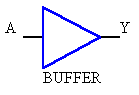Truth table:
A   Y
-----
0 = 0
1 = 1    Input signal is just buffered,
e.g. to drive more current or several other gates.Truth table:
A   Y
-----
0 = 1
1 = 0    Input signal inverted (negated)Truth table:
A B   Y
-------
0 0 = 0
0 1 = 0
1 0 = 0
1 1 = 1  Both inputs need to be HIGH to give HIGH output.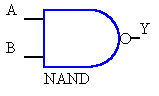Truth table:
A B   Y
-------
0 0 = 1
0 1 = 1
1 0 = 1
1 1 = 0  Both inputs need to be HIGH to give LOW output.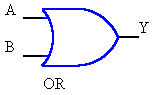Truth table:
A B   Y
-------
0 0 = 0
0 1 = 1
1 0 = 1
1 1 = 1  Both inputs need to be LOW to give LOW output,
rsp. at least 1 input must be HIGH to give HIGH output.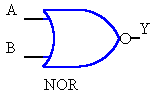Truth table:
A B   Y
-------
0 0 = 1
0 1 = 0
1 0 = 0
1 1 = 0  Both inputs need to be LOW to give HIGH output.Truth table:
A B   Y
-------
0 0 = 0
0 1 = 1
1 0 = 1
1 1 = 0  Both inputs need to be EQUAL to give LOW output.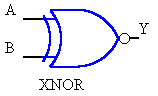Truth table:
A B   Y
-------
0 0 = 1
0 1 = 0
1 0 = 0
1 1 = 1  Both inputs need to be EQUAL to give HIGH output.

```